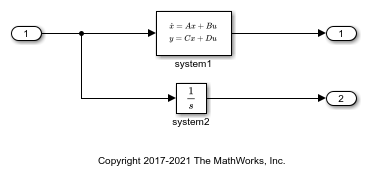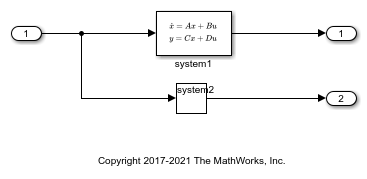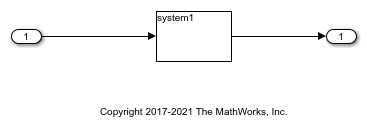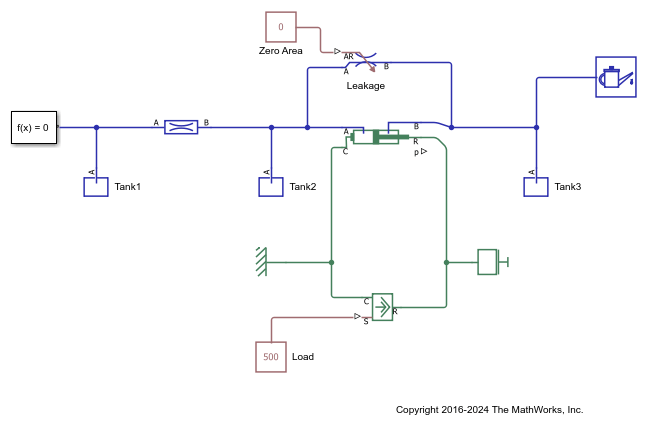Documentation

### This is machine translation

Mouseover text to see original. Click the button below to return to the English version of the page.

Note: This page has been translated by MathWorks. Click here to see
To view all translated materials including this page, select Country from the country navigator on the bottom of this page.

# getStateIndex

Get index of a state element of an operating point specification or operating point

The `States` property of an operating point specification is an array that contains trimming specifications for each model state. When defining a mapping function for customized trimming of Simulink® models, `getStateIndex` lets you obtain the index of a state specification based on the corresponding block path or state name.

When trimming Simulink models using optimization-based search, some applications require additional flexibility in defining the optimization search parameters. For such systems, you can specify custom constraints and a custom objective function. For complex models, you can define a mapping that selects a subset of the model states, inputs, and outputs to pass to the custom constraint and objective functions. For more information, see Compute Operating Points Using Custom Constraints and Objective Functions.

## Syntax

``index = getStateIndex(op,name)``
``index = getStateIndex(op,name,element)``

## Description

example

````index = getStateIndex(op,name)` returns the index of the state specification that corresponds to `name` in the `States` property of operating point specification `op`.```

example

````index = getStateIndex(op,name,element)` returns the index of the specified `element` within a state specification for a block with multiple states.```

## Examples

collapse all

Open Simulink model.

```mdl = 'scdindex1'; mdlpath = fullfile(matlabroot,'examples','slcontrol',mdl); open_system(mdlpath) ```Create an operating point specification for model.

```opspec = operspec(mdl); ```

`opspec` contains an array of state specifications for the model.

```opspec.States ```
```(1.) scdindex1/system1 spec: dx = 0, initial guess: 0 spec: dx = 0, initial guess: 0 spec: dx = 0, initial guess: 0 (2.) scdindex1/system2 spec: dx = 0, initial guess: 0 ```

Get the index of the state specification that corresponds to the system2 block.

```index2 = getStateIndex(opspec,'scdindex1/system2') ```
```index2 = 2 1 ```

`index2(1)` is the index of the state specification object for system2 in `opspec.States`. Since this block has a single state, `index2` has a single row and `index2(2)` is `1`.

If a block has multiple states, you can obtain the indices of all the states in the corresponding state specification.

```index1 = getStateIndex(opspec,'scdindex1/system1') ```
```index1 = 1 1 1 2 1 3 ```

Each row of `index1` contains the index of one state in the system2 block. For each row, the first column contains the index of the state specification in `opspec.States`. The second column contains the index of each state element within the specification.

Open Simulink model.

```mdl = 'scdindex1'; mdlpath = fullfile(matlabroot,'examples','slcontrol',mdl); open_system(mdlpath) ```Create an operating point specification for the model.

```opspec = operspec(mdl); ```

If a block has multiple states, you can obtain the index of a specific state within the corresponding state specification by specifying the element index. For example, get the index for the second state in the specification for the system1 block.

```index1 = getStateIndex(opspec,'scdindex1/system1',2) ```
```index1 = 1 2 ```

You can also obtain the indices of a subset of the block states by specifying the element index as a vector. For example, get the indices for the first and third states in the specification for the system1 block.

```index2 = getStateIndex(opspec,'scdindex1/system1',[1 3]) ```
```index2 = 1 1 1 3 ```

Open Simulink model.

```mdl = 'scdindex2'; mdlpath = fullfile(matlabroot,'examples','slcontrol',mdl); open_system(mdlpath) ```The system1 block is a state-space system with three named states: `position`, `velocity`, and `acceleration`.

Create an operating point specification for the model.

```opspec = operspec(mdl); ```

The `States` property of the operating point specification object contains one entry for each named state in system1.

```opspec.States ```
```(1.) position spec: dx = 0, initial guess: 0 (2.) velocity spec: dx = 0, initial guess: 0 (3.) acceleration spec: dx = 0, initial guess: 0 ```

To obtain the index of a state specification that corresponds to a named state within a block, specify the state name.

```index1 = getStateIndex(opspec,'velocity') ```
```index1 = 2 1 ```

The first column of `index1` contains the index of the corresponding state specification in the `opspec.States` property. The second column is `1` for a named state.

Open model.

```mdl = 'scdTanks_simscape'; mdlpath = fullfile(matlabroot,'examples','slcontrol',mdl); open_system(mdlpath) ```Create an operating point specification for the model.

```opspec = operspec(mdl); ```

The `States` property of the operating point specification object contains one state specification for each Simscape state in the model.

To obtain the index of a specification that corresponds to a Simscape state, specify the state name. For example, get the index of the pressure state of Tank3.

```idx = getStateIndex(opspec,'scdTanks_simscape.Tank3.pressure') ```
```idx = 21 1 ```

The first column of `idx` contains the index of the corresponding state specification in `opspec.States`. The second column is `1` for a Simscape state.

View the specification in `opspec.States` for this state.

```opspec.States(idx(1)) ```
```(1.) scdTanks_simscape.Tank3.pressure spec: dx = 0, initial guess: 0 ```

## Input Arguments

collapse all

Operating point specification or operating point for a Simulink model, specified as an `operspec` object or operating point object.

Block path or state name that corresponds to a state specification in the `States` property of `op`, specified as a character vector or string that contains one of the following:

• Block path of a block in the Simulink model that contains unnamed states.

• Name of a named state in a Simulink or Simscape™ block.

To see all the states that have state specifications, view the `States` property of `op`.

`op.States`

State element index, specified as a positive integer less than or equal to the number of state elements in the block or state specified by `name`, or a vector of such integers. By default, if you do not specify `element`, `getStateIndex` returns the indices of all elements in the selected state specification. For an example, see Get Index of Specified State Element of Operating Point Specification.

## Output Arguments

collapse all

State index, returned as a 2-element row vector when `element` is an integer, or a 2-column array when `element` is a vector. Each row of `index` contains the index for a single model state.

The first column of `index` contains the index of the corresponding state specification in the `States` property of `op`. The second column contains the element index within the state specification.

Using `index`, you can specify the state portion of a custom mapping for customized trimming of Simulink models. For more information, see the `CustomMappingFcn` property of `operspec`.

## See Also

#### Introduced in R2017a

##### SupportGet trial now